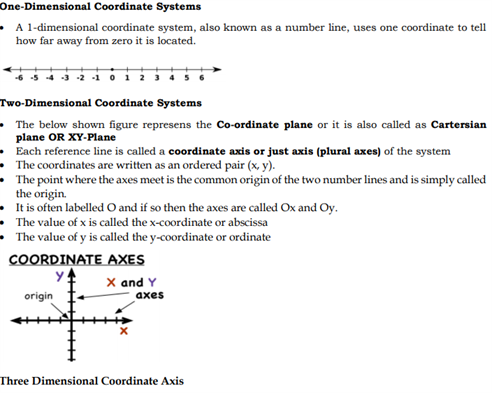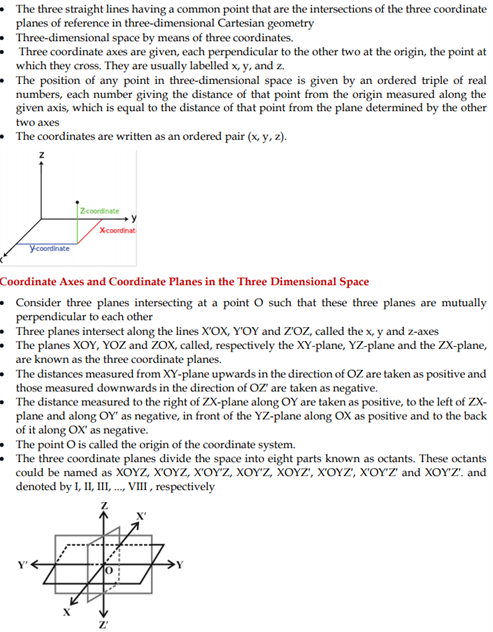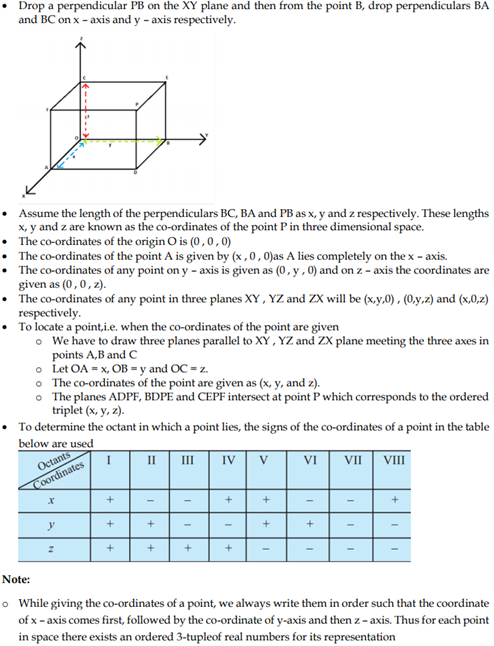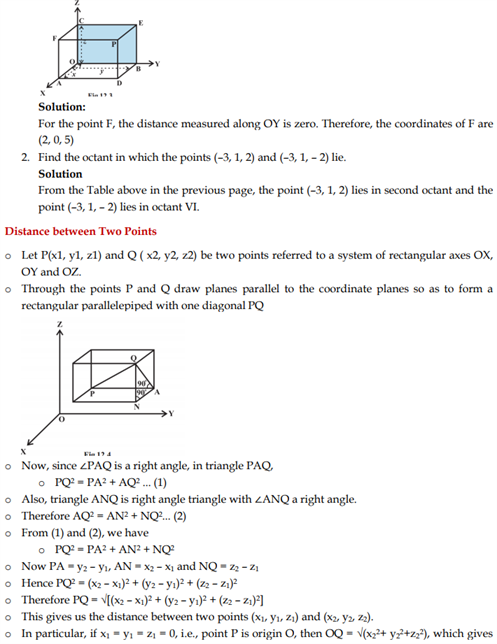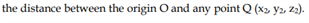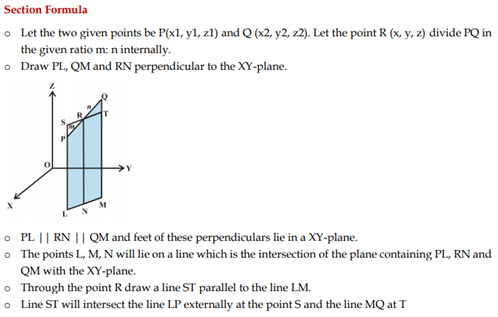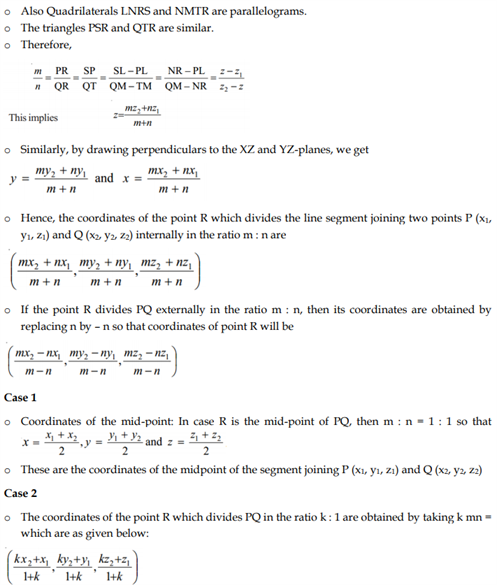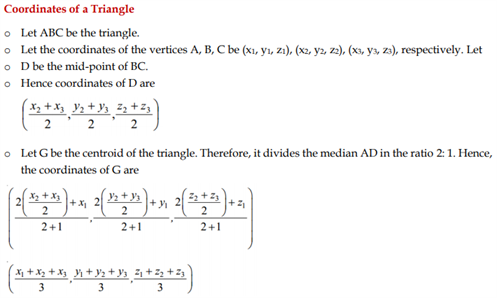# Revision Notes For CBSE Class 11 Maths Chapter 12 Introduction To 3 D Geometry

Geometry being a very important subject is a must read for getting to know the principles and formulas behind every logical explanation with respect to geometrical figures. This chapter of class 11 maths talks about the basic methods used to measure figures in one dimensional and two-dimensional plane. We know that we use vector-algebra method to study three-dimensional geometry. And the basic definition of fundamental figures is given below:

• Points are defined as the triples of real numbers arranged in an order such as P1 = (x1, y1, z1) and P2 = (x2, y2, z2)
• A Cartesian coordinate system is a coordinate system that specifies each point uniquely in a plane by a pair of numerical coordinates, which are the signed distances to the point from two fixed perpendicular directed lines, measured in the same unit of length.
• The coordinates of a point are a pair of numbers that define its exact location on a two-

dimensional plane A number on the x-axis called an x-coordinate, and a number on the y-axis called a y-coordinate.

• An ordered pair contains the coordinates of one point in the coordinate system.

The order in which you write x- and y-coordinates in an ordered pair is very important.

The x-coordinate always comes first, followed by the y-coordinate There is also a three coordinate called Z coordinate

Students would discover here the method of finding the distance between two points along with coordinates of a triangle. They would get to know the basics of geometry.

Find the maths revision notes for class 11 chapter 12 Introduction To 3 D geometry below.